## Anatomy of Timer in Structured Text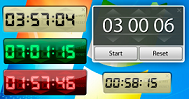Timers are used to measure specific time intervals. Timers circuits have different applications in different systems. A timer is a specialized type of clock used for measuring specific time intervals. The timer is used to generate signals for checking or doing specific or periodic task on particular time and/or with some intervals.

Now  double click on the MAIN (PRG) and it will open an editor where we can replace it with the following code (by copy paste to the upper part and lower part of the editor).

`//Header, upper partPROGRAM MAINVARtestBool : BOOL := FALSE;	testInt : INT := 0;testReal : REAL := 3.14;testString : STRING := 'MyString';myTimer : TON;END_VAR`

```//Lower part (body of the program)myTimer(IN:= TRUE, PT:=T#500MS);
IF myTimer.Q = TRUE THEN
myTimer(IN:= FALSE);
testInt := testInt +1;
IF testInt > 30999 THEN
testInt:= 0;
END_IF
END_IF```

When we make the program by using the template provided by Beckhoff, it creates a header and empty body. Now it is programmer’s work to continue from this point building the program according to the requirement.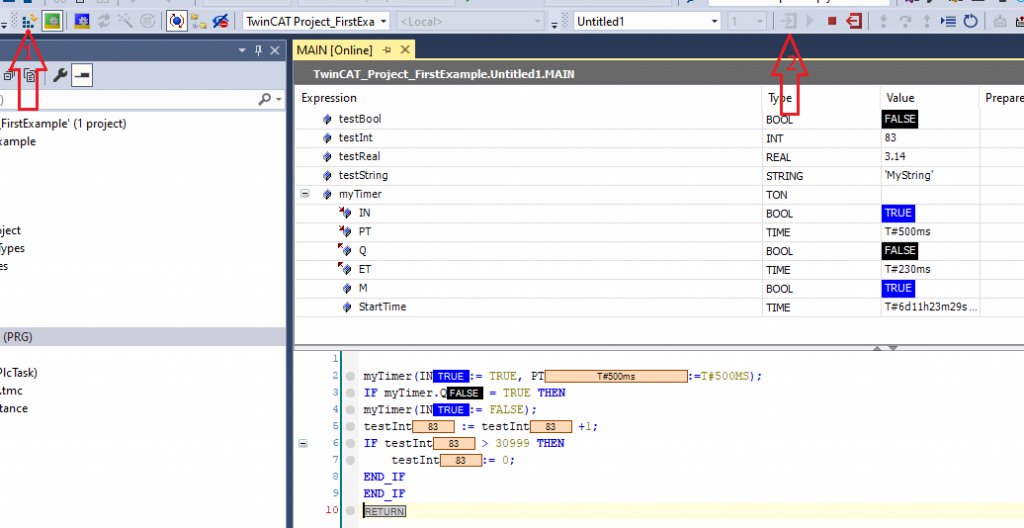If  we activate the program by pressing activate button in visual studio (arrow 1) and then login to the program (arrow 2) we see the above program running.

Congratulations! you have made your first ST program that is running in TwinCAT simulator.

Now let’s study the timer, what is happening under the hood.

`myTimer : TON; //myTimer variable of type TON, TON is a function block`

A function block is a POU (program organization unit), which returns one or several values when executed.  Typically it has input and output.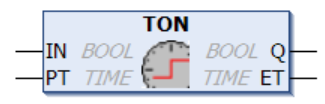Taken from Beckhoff site

If the Input IN is high then after PT milliseconds the Output Q will be high. ET will be counted in milliseconds until the Q is HIGH. Now let’s modify the above program and introduce HMI. We shall change the value of IN with  button and see how the value Q, ET and PT changes in historical view.We modify the original program a bit and insert an HMI project into the solution. If you don’t know the HMI, please take a look at https://www.hemelix.com/scada-hmi/twincat-hmi/twincat-hmi-installation/

```PROGRAM MAIN
VAR
timerToggle : BOOL := FALSE;
valuePT : REAL := 1.0;
valueQ : INT := 0;
valueET : INT := 0;
valueCounter: INT := 0;
myTimer : TON;
END_VAR```

```//Lower part (body of the program)
myTimer(IN:= timerToggle, PT:=REAL_TO_TIME(1000 * valuePT));
IF myTimer.Q = TRUE THEN
myTimer(IN:= FALSE);
valueCounter := valueCounter +1;
IF valueCounter > 30999 THEN
valueCounter:= 0;
END_IF
END_IF```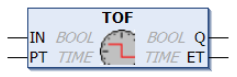Difference between TON and TOF is  that the output the output Q will be reset after PT milliseconds since IN is reset, as shown in the following figure. If you compare the timing diagram between TON and TOF then it can be clear.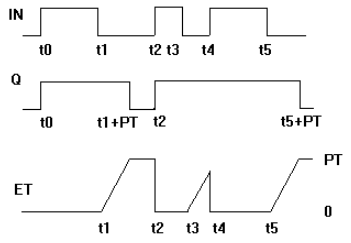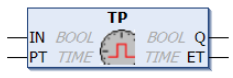The pulse timer block can be used to generate output pulses of a given time duration.

VAR_INPUT

`VAR_INPUT        IN      : BOOL; (* Trigger for Start of the Signal *)        PT      : TIME; (* The length of the High-Signal in ms *)END_VAR`

VAR_OUTPUT

`VAR_OUTPUT        Q       : BOOL; (* The pulse *)        ET      : TIME; (* The current phase of the High-Signal *)END_VAR`

If IN is FALSE, Q is FALSE and ET is 0.

As input IN goes TRUE, tho output Q follows and remains TRUE for the pulse duration as specified by time input PT.

While the pulse output ist TRUE, the elapsed time ET ist increased.

On the termination of the pulse, the elapsed time is held until the beginning of the next pulse, at which point it is reset.

The output Q will remain TRUE until the pulse time has elapsed, irrespective of the state of the input IN.

Graphic Display of the TP Time Sequence: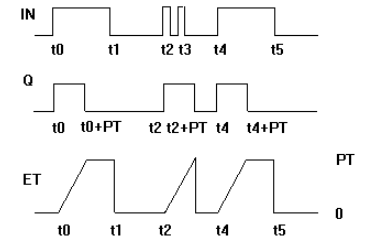## FUNCTION_BLOCK R_TRIG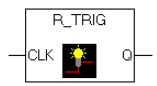Detector for a Rising Edge.

VAR_INPUT

`VAR_INPUT        CLK     : BOOL; (* Signal to detect *)END_VAR`

VAR_OUTPUT

`VAR_OUTPUT        Q       : BOOL; (* Edge detected  *)END_VAR`

The output Q and the help variable M will remain FALSE as long as the input variable CLK is FALSE. As soon as CLK returns TRUE, Q will first return TRUE, then M will be set to TRUE. This means each time the function is called up, Q will return FALSE until CLK has falling edge followed by an rising edge.

This cane be useful when we need to count some variable based on the state change of certain variable.

`PROGRAM MAINVARtimerToggle : BOOL := FALSE;timerToggle2 : BOOL := FALSE;	myTrig : R_TRIG;variable : INT := 0;END_VAR`
`//Body of the programmyTrig(CLK:= timerToggle);IF myTrig.Q THEN	variable := variable +1 ;END_IFtimerToggle2:= myTrig.M;`

Whenever the timerToggle variable goes up that is detected by myTrig.Q.  F_TRIG (falling edge) is just opposite of R_TRIG (rising edge)